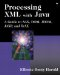# Writing XML Documents with JDOM

Once you've created a document, you're likely to want to serialize it to a network socket, a file, a string, or some other stream. JDOM's org.jdom.output.XMLOutputter class does this in a standard way. You can create an XMLOutputter object with a no-args constructor and then write a document onto an OutputStream with its output() method. For example, the following code fragment writes the Document object named doc onto System.out :

` XMLOutputter outputter = new XMLOutputter();  try {   outputter.output(doc, System.out); } catch (IOException e) {   System.err.println(e); } `

Although you also can output a document onto a java.io.Writer , it's recommended that you use an OutputStream because it's generally not possible to determine the underlying encoding of a Writer and set the encoding declaration accordingly .

In addition to documents, XMLOutputter can write elements, attributes, CDATA sections, and all of the other JDOM node classes. For example, the following code fragment writes an empty element named Greeting onto System.out :

` XMLOutputter outputter = new XMLOutputter();  try {   Element element = new Element("Greeting");   outputter.output(element, System.out); } catch (IOException e) {   System.err.println(e); } `

This may be useful occasionally; but if you write anything other than a single Document or Element onto a stream, the result probably won't be a well- formed XML document.

Finally, instead of writing onto a stream or writer, you can use the outputString() methods to store an XML document or node in a String . This is often useful when passing XML data through non-XML-aware systems. For example, the following code fragment stores an empty element named Greeting in the String variable named hello :

` XMLOutputter outputter = new XMLOutputter();  Element element = new Element("Greeting"); String hello = outputter.outputString(element); `

Example 14.1 puts this all together with a simple program that generates the Fibonacci series in XML format.

##### Example 14.1 A JDOM Program That Produces an XML Document Containing Fibonacci Numbers
` import org.jdom.*; import org.jdom.output.XMLOutputter; import java.math.BigInteger; import java.io.IOException; public class FibonacciJDOM {   public static void main(String[] args) {     Element root = new Element("Fibonacci_Numbers");     BigInteger low  = BigInteger.ONE;     BigInteger high = BigInteger.ONE;     for (int i = 1; i <= 5; i++) {       Element fibonacci = new Element("fibonacci");       fibonacci.setAttribute("index", String.valueOf(i));       fibonacci.setText(low.toString());       root.addContent(fibonacci);       BigInteger temp = high;       high = high.add(low);       low = temp;     }     Document doc = new Document(root);     // serialize it onto System.out     try {       XMLOutputter serializer = new XMLOutputter();       serializer.output(doc, System.out);     }     catch (IOException e) {       System.err.println(e);     }   } } `

The output is as follows :

` D:\books\XMLJAVA\examples>  java   FibonacciJDOM  <?xml version="1.0" encoding="UTF-8"?> <Fibonacci_Numbers><fibonacci index="1">1</fibonacci><fibonacci index="2">1</fibonacci><fibonacci index="3">2</fibonacci> <fibonacci index="4">3</fibonacci><fibonacci index="5">5 </fibonacci></Fibonacci_Numbers> `

This isn't especially pretty, but there are a couple of ways to clean it up. First off you can recognize that white space is significant in XML, and by default JDOM faithfully reproduces it. Thus if you want the output to be indented, you could add strings containing line breaks and extra space in the right places. Alternatively, if you happen to know that white space is not significant in the particular XML vocabulary the program writes, then you can ask the XMLOutputter to format the document for you. For example, this XMLOutputter inserts the default line ending after elements and indents elements by two spaces per each layer of the hierarchy:

` XMLOutputter serializer = new XMLOutputter();  serializer.setIndent("  "); // use two space indent serializer.setNewlines(true); serializer.output(doc, System.out); `

Now the output looks like this:

` <?xml version="1.0" encoding="UTF-8"?>  <Fibonacci_Numbers>   <fibonacci index="1">1</fibonacci>   <fibonacci index="2">1</fibonacci>   <fibonacci index="3">2</fibonacci>   <fibonacci index="4">3</fibonacci>   <fibonacci index="5">5</fibonacci> </Fibonacci_Numbers> `

Much prettier, I think you'll agree.

You can also specify the amount of indenting to use and whether or not to add line breaks as arguments to the XMLOutputter() constructor, as follows:

` XMLOutputter serializer = new XMLOutputter("  ", true);  serializer.output(doc, System.out); `

For another example, let's revisit FlatXMLBudget in Example 4.2. Recall that its purpose was to read a tab-delimited file containing financial data and convert it into XML. The method that actually generated the XML was convert() , and it did this by writing strings onto an OutputStream like so:

` public static void convert(List data, OutputStream out)   throws IOException {   Writer wout = new OutputStreamWriter(out, "UTF8");   wout.write("<?xml version=\"1.0\"?>\r\n");   wout.write("<Budget>\r\n");   Iterator records = data.iterator();   while (records.hasNext()) {     wout.write(" <LineItem>\r\n");     Map record = (Map) records.next();     Set fields = record.entrySet();     Iterator entries = fields.iterator();     while (entries.hasNext()) {       Map.Entry entry = (Map.Entry) entries.next();       String name = (String) entry.getKey();       String value = (String) entry.getValue();       // some of the values contain ampersands and less than       // signs that must be escaped       value = escapeText(value);       wout.write("    <" + name +">");       wout.write(value);        wout.write("</" + name +">\r\n");     }     wout.write("  </LineItem>\r\n");   }   wout.write("</Budget>\r\n");   wout.flush(); } `

JDOM can make this method quite a bit simpler, as well as completely eliminate the need for the escapeText() method, since JDOM handles that internally:

` public static void convert(List data, OutputStream out)   throws IOException {   Element budget = new Element("Budget");     Iterator records = data.iterator();   while (records.hasNext()) {     Element lineItem = new Element("LineItem");     budget.addContent(lineItem);     Map record = (Map) records.next();     Set fields = record.entrySet();     Iterator entries = fields.iterator();     while (entries.hasNext()) {       Map.Entry entry = (Map.Entry) entries.next();       String name = (String) entry.getKey();       String value = (String) entry.getValue();       Element category = new Element(name);       category.setText(value);       lineItem.addContent(category);     }   }   Document doc = new Document(budget);   XMLOutputter outputter = new XMLOutputter("  ", true);   outputter.output(doc, out);   out.flush(); } `

The disadvantage to this approach is that even though the input is streamed, the output is not. The entire document is built and stored in memory before the first byte of output is written. This can be a problem in devices with limited memory or with large documents.Processing XML with Javaв„ў: A Guide to SAX, DOM, JDOM, JAXP, and TrAX
ISBN: 0201771861
EAN: 2147483647
Year: 2001
Pages: 191